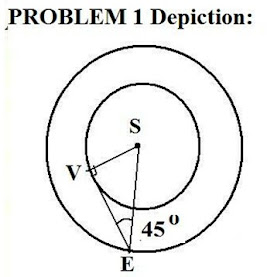## Friday, January 14, 2022

### Solutions To Basic Astronomical Trig Problems

1) Estimate the distance of Venus from the Sun at its most recent maximum elongation, if the angle of max. elongation was 46 degrees.

Solution:

The diagram for Problem 1 is shown below, including the angle of maximum elongation:We know, from the geometry:

SV/ SE = sin (SEV)

Then Venus' distance is:

SV = (sin(SEV)) SE

where: angle SEV = 46 deg and SE = a(E) = 1 AU

then:

SV = sin (46) 1 AU = (0.719) 1 AU = 0.719 AU

2) A recent observation of Mars 36.5 days after opposition showed an angle of elongation = 136 degrees. Find the distance of Mars from the Earth if Mars' orbital period = 687 days.

Solution:

The diagram for the configuration is shown below:

We are given t = 36.5 d, and we need to find the "gain" angle:

Θ = 360 (1/P - 1/P') t

where P, P' are the sidereal periods, or P = 365.25 d (Earth) and P' = 687 d (Mars)

Then:

Θ = 360 (1/365.25 - 1/687) (36.5) = 16.o8

which is none other than angle E1Sp1.

Now, angle E1p1S is required in order to get the distance - as the information at the bottom of the depiction shows.

We know:

Ep1S = 180 - [SE1p1 - E1Sp1]

and SE1p1 = 136 o

So:

Ep1S = 180 - [136 o - 16. o 8 ]= 27. o 2

Again, from plane trigonometry:

sin(p1E1S)/ Sp1 = sin(E1p1S)/ SE1

and, the ratio of planetary distances at time t:

Sp1/SE1 = sin (p1E1S)/ sin (E1p1S)

But:

E1p1S = 27. o2

and p1E1S = SE1p1 = 136 o

Thus:

Sp1/SE1 = sin (136)/ sin (27.2)

Sp1 = (0.694/ 0.457) a(E) = 1.518 (a(E)) = 1.518 AU